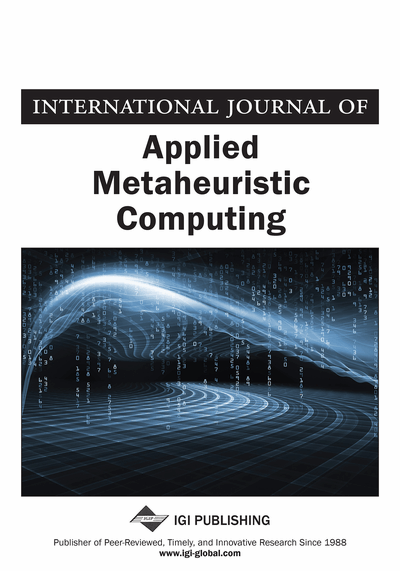# A Hybrid Particle Swarm Optimization Method for Traveling Salesman Problem

Yong Wang, Ning Xu
DOI: 10.4018/IJAMC.2017070104
OnDemand:
(Individual Articles)
Available
\$29.50
No Current Special Offers

## Abstract

Traveling salesman problem (TSP) is one well-known NP-Complete problem. The objective is to search the optimal Hamiltonian circuit (OHC) in a tourist map. The particle swarm optimization (PSO) integrated with the four vertices and three lines inequality is introduced to detect the OHC or approximate OHC. The four vertices and three lines inequality is taken as local heuristics to find the local optimal paths composed of four vertices and three lines. Each of this kind of paths in the OHC or approximate OHC conforms to the inequality. The particle swarm optimization is used to search an initial approximation. The four vertices and three lines inequality is applied to convert all the paths in the approximation into the optimal paths. Then a better approximation is obtained. The method is tested with several Euclidean TSP instances. The results show that the much better approximations are searched with the hybrid PSO. The convergence rate is also faster than the traditional PSO under the same preconditions.
Article Preview
Top

## 1. Introduction

Traveling salesman problem (TSP) is one of well-known NP-Complete problems. It aims to find the optimal Hamiltonian circuit (OHC) in a given tourist map. The circuit with each of the cities once and exactly once is called a Hamiltonian circuit (HC). The OHC is the shortest HC among all of the HCs. However, the number of the HCs increases exponentially in proportion to the number of the cities (Rodríguez and Ruiz, 2012). It is impossible to find the OHC through traversing all of the HCs. TSP has been widely studied in the fields of computer science, combinational mathematics, and graph theory. Many industrial applications, such as the network optimization, vehicle path planning, manufacturing process planning, etc. have close relationships with TSP. The efficient methods are urgently advocated to resolve them within an acceptable computation time.

At first, the exact algorithms are designed for TSP. The OHC is guaranteed to find with the exact algorithms. These algorithms include the graph-search algorithms (Douglas, 2006), integer programming and dynamic programming methods. The cutting-plane and branch-and-bound methods are two kinds of efficient algorithms for the TSP with a few hundred cities (Gouveia and Voβ, 1995; Klerk and Dobre, 2011). For the large scale of TSP with thousands of cities, the computation time is too long. In addition, the powerful computers are usually applied to search the optimal solutions based on the exact algorithms (Applegate, et al., 2009). Through decades of research, it seems that there is no polynomial algorithm for the hard problem unless P = NP. It is an open problem that the existence of a polynomial algorithm for TSP.

The approximate algorithms cannot guarantee to search the OHC whereas the time complexity of them is polynomial. The approximate algorithms play an important role to cope with the complex TSP. Most of the approximate algorithms search an approximation within a reasonable computation time. The local heuristics (Bläser, 2008; Bontoux et al., 2010) are widely used to enhance their performance. The approximate algorithms for the TSP include the (nearest) neighborhood algorithms (Li et al, 2011), minimum spanning tree algorithm and the Christofides’ algorithm, etc. (Johnson and McGeoch, 2004). The k-opt (k = 2, 3, 4, 5) methods and the following LKH algorithms derived from them are taken as one of the most competitive algorithms for TSP (Helsgaun, 2012). The research illustrates that they are robust for large TSP with thousands of cities. The approximate algorithms are always improved and their performance is enhanced gradually. For the large scale of TSP, the solutions quality searched with the approximate algorithms are hard to evaluate because the OHC is not known.

Except for the exact and approximate algorithms, the intelligent optimization algorithms are extensively applied to resolve TSP. The intelligent optimization algorithms find the optimal or approximate solutions with respect to the evolutionary rules. They usually find the approximations in most cases. These algorithms include the genetic algorithms (Liu, 2010), the heuristic neural network (Ghaziri and Osman, 2003), the ACO (Dorigo, et al., 2006; Zhou, 2009), the simulated annealing algorithm (Liu et al., 2009), the particle swarm optimization algorithm (Chen et al., 2010) and the consultant-guided search algorithm (Iordache, 2010), etc. Different from the local heuristics used in the approximate algorithms, the intelligent optimization algorithms transform the HCs into the OHC or approximate OHC based on the evolutionary rules controlled by their random parameters. The approximate algorithms (Lin-Kernighan) with the intelligent algorithms (generic algorithm) are researched to enhance the performance of the hybrid algorithms (Bontoux et al., 2010; Baraglia, 2001). The intelligent algorithms show good performance to resolve the complex optimization problems whereas they are apt to trap into the local minima. Therefore, the improvements of these algorithms are always concentrated on.

## Complete Article List

Search this Journal:
Reset
Volume 14: 1 Issue (2023)
Volume 13: 4 Issues (2022): 2 Released, 2 Forthcoming
Volume 12: 4 Issues (2021)
Volume 11: 4 Issues (2020)
Volume 10: 4 Issues (2019)
Volume 9: 4 Issues (2018)
Volume 8: 4 Issues (2017)
Volume 7: 4 Issues (2016)
Volume 6: 4 Issues (2015)
Volume 5: 4 Issues (2014)
Volume 4: 4 Issues (2013)
Volume 3: 4 Issues (2012)
Volume 2: 4 Issues (2011)
Volume 1: 4 Issues (2010)
View Complete Journal Contents Listing